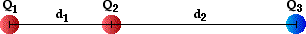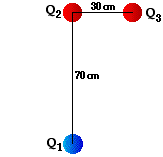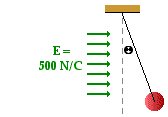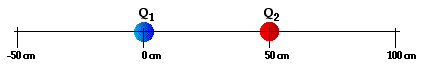# Electricity: Static Electricity

## Static Electricity: Problem Set

Use 8.99 x109 N•m2/C2 for Coulomb's constant and 1.60x10-19 C for the charge of an electron.

#### Problem 1:

Determine the quantity of charge on …
a. … a plastic tube which has been rubbed with animal fur and gained 3.8x109 electrons.
b. … a vinyl balloon which has been rubbed with animal fur and gained 1.7x1012 electrons.
c. … an acetate strip which has been rubbed with wool and lost 7.3x108 electrons.

#### Problem 2:

A 250 mL aqueous solution contains 2.37 g of copper(II) chloride. The dissolved copper(II) chloride is dissociated into copper(II) and chloride ions. Each copper(II) ion has two less electrons than protons. A scientist wishes to use an electroplating process to reduce the 1.12 g of copper ions to solid copper atoms. To be reduced from the ion form to the atom form, a copper(II) ion must gain two electrons. Each gram of copper(II) ions contains 9.48 x 1021 ions. Determine the total quantity of charge that must be supplied to turn the copper(II) ions into solid copper atpms.

#### Problem 3:

Two ping pong balls have been painted with metallic paint and charged by contact with an Van de Graaff generator. The charge on the balls are -3.1x10-7 C and -3.7x10-7 C. Determine the force of electrical repulsion when held a distance of 42 cm apart.

#### Problem 4:

A Styrofoam plate with a negative charge of -4.86x10-7 C is placed near an aluminum dish which has been charged positively by induction to a charge of +8.29x10-8 C. The centers of positive and negative charge are positioned 1.85 cm apart. Determine the magnitude of the force of attraction between the Styrofoam plate and the aluminum dish.

#### Problem 5:

Let's just suppose that Tyrone transferred a Coulomb of negative charge to Mia so that Tyrone had a +1.0 C charge and Mia had a -1.0 C charge. Determine the force of electrical attraction between Tyrone and Mia if they are positioned …
a. … in their seats with a separation distance of 1.0 m.
b. … in South's physics classroom and at Navy Pier 52 km away.
c. … in South's physics classroom and Disney World in Orlando, Florida 1900 km away.

#### Problem 6:

Mr. H gives two large vinyl balloons ten good rubs on what's left of his hair, transferring a total of 2.1x1012 electrons from his hair to each balloon. He walks away, leaving the balloons to be held by strings from a single pivot point on the ceiling. The balloons repel and reach an equilibrium position with a separation distance of 58 cm.
a. Determine the quantity of charge on each balloon.
b. Determine the Coulomb force of repulsion between the two balloons.

#### Problem 7:

It happened during Wednesday's physics lesson on charging by friction. A small white object began to slowly emerge from beneath the sleeve of Mr. H's sweater. It took several laughs and giggles from the class before the emerging sheet of fabric softener caught Mr. H's attention. He turned the potentially embarrassing moment into a serendipitous moment. Pulling the sheet out from beneath the sweater, Mr. H wrote the following problem on the board.

A sheet of cationic fabric softener having a surface charge of 3.6x10-12 C (positive) clings to a wool sweater with a negative charge of 6.8x10-9 C. Determine the force of electrical attraction if they can be treated as point objects with a separation distance of 0.019 cm.

What is the answer to this problem?

#### Problem 8:

Two vinyl balloons with an identical charge are given a separation distance of 52 cm. The balloons experience a repulsive force of 2.74x10-3 N. Determine the magnitude of charge on each one of the balloons.

#### Problem 9:

Two different objects are given charges of +3.27 μC and -4.91 μC. What separation distance will cause the force of attraction between the two objects to be 0.358 N? (GIVEN: 1 C = 106 μC)

#### Problem 10:

What mass would a pair of electrons have in order for their gravitational force of attraction to be equal to the electrical force of repulsion? (Use G=6.67x10-11 N•m2/kg2.)

#### Problem 11:

An electron has a mass of 9.11x10-31 kg. In the Bohr model of the hydrogen atom, the electron was viewed as orbiting the lone proton of the nucleus; the centripetal force requirement was met by the electrical attraction between the oppositely charged proton and electron. The radius of orbit was 5.29x10-11 m. Use circular motion and electrostatic principles to determine the speed at which the electron moves as it orbits the proton.

#### Problem 12:

Two bowling balls with a mass of 7.25 kg are positioned in a vertical cylinder such that they fit one above the other. What quantity of charge must the two bowling balls have such that the electrical force of repulsion causes the top ball to levitate above the bottom ball such that their nearest surfaces are distanced 25 cm apart? The diameter of a bowling ball is 21.8 cm. Assume the bowling balls have an identical charge.

#### Problem 13:

Mr. H rubs a long plastic tube with wool and imparts a charge of -45.6 nC to the tube. Ariel then blows a soap bubble into the air. Mr. H holds the plastic tube above the bubble, causing it to morph into an oblong shape and suspend motionless just below the tube. As the 22.8-mg bubble polarizes, the center of positive charge of the bubble is positioned 11.4 cm from the charge on the plastic tube; the center of negative charge is positioned 16.9 cm from the charge on the plastic tube. Ignoring buoyant forces, determine the effective positive and negative charge on the polarized bubble. (GIVEN: 1 C = 109 nC)

#### Problem 14:

Two objects with charges Q1 and Q2 experience an electrical force of attraction of 8.0x10-4 N when separated by a distance of d. Determine the force of attraction if the same objects are separated by …
a. … a distance of 2•d.
b. … a distance of 3•d.
c. … a distance of 0.5•d.
d. … a distance of 2d and each object having double the charge.

#### Problem 15:

The electric field intensity at a particular location surrounding a Van de Graaff generator is 4.5x103 N/C. Determine the magnitude of the force which this field would exert upon …
a. … an electron when positioned at this location.
b. … a charged balloon with 1.8 μC of charge when positioned at this location.
c. … a pith ball with 6.8x10-8 C of charge when positioned at this location.

#### Problem 16:

There is an electric field in the atmosphere surrounding the Earth which has a magnitude of roughly 150 N/C and a direction pointing towards the center of the Earth. Determine the magnitude and the direction of the force experienced by a dust particle having a negative charge of 9.2x10-16 C.

#### Problem 17:

High voltage electrical cables generate an electric field in the region of space surrounding the cables. This field can be strong enough to cause a coronal glow in grounded objects and to ignite combustible fuels. According to one source, utility companies take measures to insure that the electrical field intensity is no greater than 1000 N/C at the perimeter of their public lands. Determine the force that would be experienced by a 1.0 μC charge when placed at this location.

#### Problem 18:

A test charge with a negative charge of 2.18x10-8 C experiences a northward force of 4.50x10-5 N when placed a distance of 25.0 cm from a source charge.
a. Determine the magnitude and direction of the electric field at this location.
b. Determine the magnitude and type of charge on the source.
c. Determine the strength of the electric field at a distance of 75.0 cm from the source.

#### Problem 19:

A small balloon with a 4.36x10-9 C charge is placed a distance of 41 cm from a 1.28 μC charge.
a. Determine the electric field intensity at the location where the balloon is.
b. Determine the electrical force experienced by the balloon at this location.

#### Problem 20:

Dry air is generally a good insulator. It’s insulating ability breaks down when the electric field approaches a value of approximately 3x106 N/C. Determine the quantity of charge on a Van de Graaff generator that will produce an electric field strength of 3.0x106 N/C at a distance of 50. cm from the generator. Treat the generator as a point charge.

#### Problem 21:

The electric field between the plates of the cathode ray tube of an older television set can be as high as 2.5x104 N/C. Determine the force and resulting acceleration of an electron (m = 9.11x10-31 kg) as it travels through this electric field towards the television screen.

#### Problem 22:

Mr. H inflates a 1.4-gram balloon and charges it negatively by rubbing it on his head. He then rubs a plastic tube with animal fur to impart a charge of -4.3x10-8 C. By holding the plastic tube at a position of 12 cm below the balloon, he is able to levitate the balloon. Consider the two objects to be point charges and determine the quantity of charge upon the balloon.

#### Problem 23:Two identical balloons are inflated and charged in the same manner. They are tied by threads and hung from the same pivot point on the ceiling. The balloons hang down, with the threads making an angle of 14° with each other and the balloons being separated by a distance of 58 cm (center-to-center). Each balloon has a charge of -3.5x10-7 C. Draw a free-body diagram for the balloons and consider them to act as point objects.
a. Calculate the force of electrical repulsion between the balloons.
b. What is the horizontal component of force in the thread that supports either one of the balloons?
c. What is the vertical component of force in the thread that supports either one of the balloons?
d. What is the mass of either one of the balloons?

#### Problem 24:

Consider the diagram below.GIVEN: Q1 = +5.00x10-7 C; Q2 = +4.00x10-7 C; Q3 = -8.00x10-7 C; d1 = 5.00 cm; d2 = 8.00 cm.
a. Determine the magnitude and direction of the force exerted by Q2 upon Q1.
b. Determine the magnitude and direction of the force exerted by Q3 upon Q1.
c. Determine the magnitude and direction of the net electric force on Q1.

#### Problem 25:Three charges are arranged as shown in the diagram at the right.

GIVEN:
Q1 = -15 nC
Q2 = +14 nC
Q3 = +11 nC
1 nC = 1 nanoCoulomb = 1x10-9 C

a. Determine the magnitude and direction of the force exerted by Q1 upon Q2.
b. Determine the magnitude and direction of the force exerted by Q3 upon Q2.
c. Determine the magnitude and direction of the net electrostatic force on Q2.

#### Problem 26:

Determine the strength of the net electric field at a location midway between two point charges. The charges are Q1 = +8.32x10-9 C and Q2 = +6.04x10-9 C. The separation distance is 24.6 cm. Suggestion: construct a diagram of the arrangement of two charges and compute each individual electric field; then sum to determine the net electric field.

#### Problem 27:

Two charges are separated by a distance of 40 cm. Q1 has a charge of -4.5 nC and Q2 has a charge of +9.1 nC. (GIVEN: 1 C = 109 nC.)Determine the location where the net electric field is 0 N/C. Express your answer as an x-coordinate location (in cm), with Q1 being located at x=0 cm and Q2 being located at x = +40 cm (as shown in the diagram).

#### Problem 28:A thread is tied to a 2.50-gram pith ball and attached to the ceiling at a location of a uniform electric field with an intensity of 5.00x102 N/C. The pith ball experiences a force which causes it to deflect from an otherwise vertical alignment by an angle of 12.6° from the vertical. Use a free-body diagram and electrostatic principles to perform the following calculations.
a. Determine the force of gravity acting upon the pith ball.
b. Determine the vertical component of the tension force of the thread.
c. Determine the horizontal component of the tension force of the thread.
d. Determine the quantity of charge on the pith ball.
e. What type of charge - positive or negative - does the pith ball possess?

#### Problem 29:

Two negatively-charged balloons with a mass of 1.78 g are suspended by threads from a common point on the ceiling. The two balloons repel to an equilibrium position in which the angle between the two threads is 13.1 degrees. The distance from ceiling to the center of either one of the balloons is 155 cm. Determine the charge on each balloon. Assume the same amount of charge is on each balloon.

#### Problem 30:Four positive charges are positioned in such a manner than they form a square with sides which are 5.0 cm long. A 3.0 μC charge is placed at the (0 cm, 0 cm) mark. A 9.0 μC charge is placed at the (0 cm, 5 cm) mark, a 3 μC charge is placed at the (5 cm, 5 cm) mark, and a 6.0 μC charge is placed at the (5 cm, 0cm) mark. Determine the magnitude and direction of the electric field at the exact center of the square.

#### Problem 31:The diagram at the right shows three charges positioned to form an equilateral triangle. Each side has a length of 46 cm and each charge has a positive charge of 7.8 nC. Determine the magnitude and direction of the net electric force exerted upon the charge at point P at the top of the triangle.

#### Problem 32:

Two point charges (Q1 and Q2) are positioned along the x-axis as shown below.They are spaced 50. cm apart and have charges of +38 nC (Q1) and -18 nC (Q2). Determine the location along the axis where the net electric field is 0 N/C.

#### Problem 33:Three negative charges are arranged as shown in the diagram at the right. The charges of the three objects are:

Q1 = -6.2 nC
Q2 = -3.8 nC
Q3 = -5.5 nC
(1 nC = 1 nanoCoulomb = 1x10-9 C)

Determine the magnitude of the net electric force exerted on Q3.